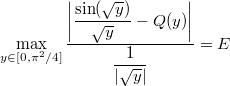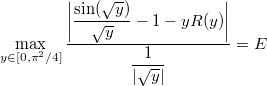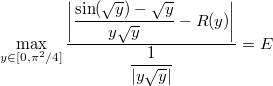Version 6 (modified by sam, 9 years ago) (diff)

fix tutorial for upcoming LolRemez 0.2

# Remez tutorial 4/5: fixing lower-order parameters

In the previous section, we took advantage of the symmetry of sin(x) to build the following minimax expression:Leading to the following coefficient, amongst others:

const double a1 = 9.999999765898820673279342160490060830302e-1;


This is an interesting value, because it is very close to 1. Many CPUs can load the value 1 very quickly, which can be a potential runtime gain.

## The brutal way

Now we may wonder: what would be the cost of directly setting a1 = 1 here? Let’s see the error value:## The clever way

The clever way involves some more maths. Instead of looking for polynomial Q(y) and setting Q(0) = 1 manually, we search instead for R(y) where Q(y) = 1 + yR(y):Dividing by y up and down gives:Once again, we get a form suitable for the Remez algorithm.

## Source code

#include "lol/math/real.h"
#include "lol/math/remez.h"

using lol::real;
using lol::RemezSolver;

real f(real const &y)
{
real sqrty = sqrt(y);
return (sin(sqrty) - sqrty) / (y * sqrty);
}

real g(real const &y)
{
return re(y * sqrt(y));
}

int main(int argc, char **argv)
{
RemezSolver<3, real> solver;
solver.Run("1e-1000", real::R_PI_2 * real::R_PI_2, f, g, 40);
return 0;
}


Only f and g changed here, as well as the polynomial degree. The rest is the same as in the previous section.

## Compilation and execution

Build and run the above code:

make
./remez


After all the iterations the output should be as follows:

Step 8 error: 4.618689007546850899022101933442449327546e-9
Polynomial estimate:
x**0*-1.666665709650470145824129400050267289858e-1
+x**1*8.333017291562218127986291618761571373087e-3
+x**2*-1.980661520135080504411629636078917643846e-4
+x**3*2.600054767890361277123254766503271638682e-6


We can therefore write the corresponding C++ function:

double fastsin2(double x)
{
const double a3 = -1.666665709650470145824129400050267289858e-1;
const double a5 = 8.333017291562218127986291618761571373087e-3;
const double a7 = -1.980661520135080504411629636078917643846e-4;
const double a9 = 2.600054767890361277123254766503271638682e-6;

return x + x*x*x * (a3 + x*x * (a5 + x*x * (a7 + x*x * a9))));
}


Note that because of our change of variables, the polynomial coefficients are now a3, a5, a7

## Analysing the results

Let’s see the new error curve:Excellent! The loss of precision is clearly not as bad as before.

## Conclusion

You should now be able to fix lower-order coefficients in the minimax polynomial for possible performance improvements.

Please report any trouble you may have had with this document to sam@hocevar.net. You may then carry on to the next section: additional tips.